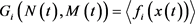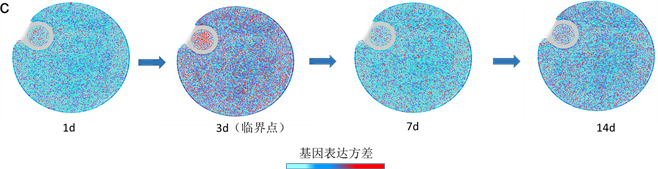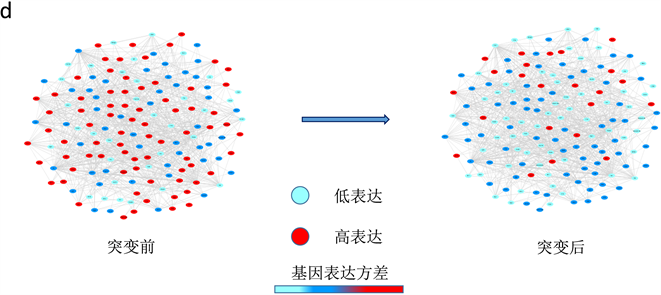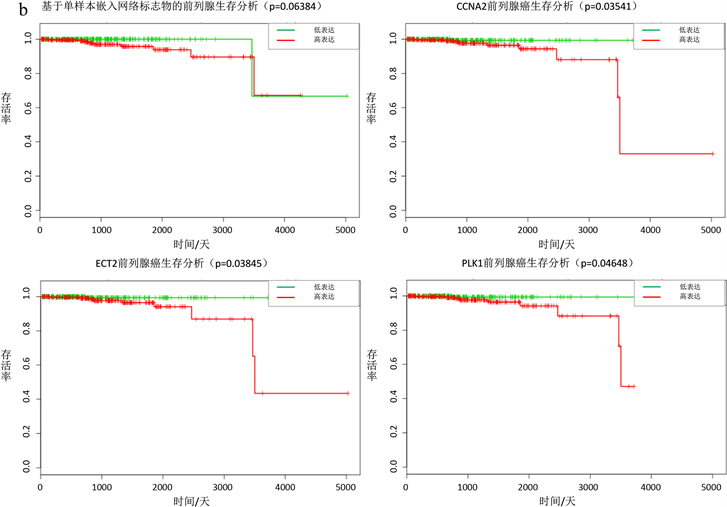基于个体特定网络和矩升维降噪法来探测疾病系统临界信号 Detection of Critical Signals in Disease Systems Based on Individual Specific Networks and Moment Expansion Noise Re-duction Method

• 全文下载: PDF(3226KB)    PP.70-79   DOI: 10.12677/HJCB.2018.84009
• 下载量: 161  浏览量: 242

In the process of mining biological disease data, it is often disturbed by strong noise, which makes data mining inaccurate. At this time, some traditional methods for mining critical point signals are invalid. In this paper, we adopt the method of probability distribution embedding based on individual specific network to study the two time series data of liver cancer and prostate cancer, detect the critical signal of malignant mutation of disease, and then predict the mutation point of disease. The theoretical basis of this work is to transform the big noise data of the sample state of the original system into the small noise data of the sample probability distribution by the probability distribution embedding transformation, and then establish the individual specific network. It is found that it can reduce interference of data noise and solve the problem of fewer sample data. Then, based on the dynamic network biomarkers to detect the signal of disease mutation, the functional analysis of these biomarkers shows that they can well reflect the critical signal.

1. 引言

2. 方法

2.1. 概率分布嵌入法

$\frac{\text{d}x\left(t\right)}{\text{d}t}=f\left(x\left(t\right)\right)+\eta \left(t\right)$ (1)

$\frac{\text{d}m\left(t\right)}{\text{d}t}=g\left(m\left(t\right)\right)+ϵ\left(t\right)$ (2)

$\frac{\partial P\left(X;t\right)}{\partial t}=\underset{k=1}{\overset{n}{\sum }}\left[{\omega }_{k}\left(X-{\theta }_{k}\right)P\left(X-{\theta }_{k};t\right)-{\omega }_{k}\left(X\right)P\left(X;t\right)\right]$ (3)

$\frac{\partial P\left(X\left(t\right);t\right)}{\partial t}=\underset{k=1}{\overset{n}{\sum }}\left[-\underset{i=1}{\overset{m}{\sum }}{\theta }_{k,i}\frac{\partial }{\partial {X}_{i}}+\underset{i,j=1}{\overset{m}{\sum }}\frac{{\theta }_{k,i}{\theta }_{k,j}}{2}\frac{{\partial }^{2}}{\partial {X}_{i}\partial {X}_{j}}\right]\left(\omega \left(X\left(t\right)\right)P\left(X\left(t\right);t\right)\right)$ (4)

$\frac{\text{d}{X}_{i}\left(t\right)}{\text{d}t}={F}_{i}+{\xi }_{i}\left(t\right)$ (5)

$\frac{\text{d}〈g\left(X\left(t\right),s\right)〉}{\text{d}t}=〈\underset{i=1}{\overset{m}{\sum }}\text{ }{F}_{i}\frac{\partial g}{\partial {X}_{i}}〉+\underset{i,j=1}{\overset{m}{\sum }}〈{F}_{i,j}\frac{{\partial }^{2}g}{\partial {X}_{i}\partial {X}_{j}}〉$ (6)

$\frac{\text{d}{N}_{i}\left(t\right)}{\text{d}t}={G}_{i}\left(N\left(t\right),M\left(t\right)\right)$ (7)

$\frac{\text{d}{M}_{ij}\left(t\right)}{\text{d}t}={G}_{ij}\left(N\left(t\right),M\left(t\right)\right)$ (8)(9)

${G}_{ij}\left(N\left(t\right),M\left(t\right)\right)=〈\left({x}_{i}\left(t\right)-{N}_{i}\left(t\right)\right){f}_{j}\left(x\left(t\right)\right)+\left({x}_{j}\left(t\right)-{N}_{j}\left(t\right)\right){f}_{i}\left(x\left(t\right)\right)〉+{\sigma }_{ij}$ (10)

2.2. 个体特定网络

(A)中(a, b)表示单变量动态系统在不同噪声下随着参数或时间的表现行为。(a)表示单变量系统在小噪声下时，临界点和系统分岔点位置非常接近，此时传统方法(如临界慢化方法)可以检测预警信号。(b)表Figure 1. Model of probability distribution embedding and individual specific network

2.3. 个体特定网络概率嵌入法动态网络标志物综合指标

1) 显著组中的每个变量的表达均值差的绝对值( $\Delta ED$ )明显增加；

2) 显著组中的变量之间的皮尔逊相关系数差的绝对值( $\Delta PC{C}_{in}$ )明显增加；

3) 显著组中的变量与非显著组中的变量之间的皮尔逊相关系数差绝对值( $\Delta PC{C}_{out}$ )明显增加。

$\Delta ISCI=\frac{\Delta ED×\Delta PC{C}_{in}}{\Delta PC{C}_{out}+\epsilon }$ (11)

3. 基于个体特定网络概率嵌入法算法流程

$N=\frac{{D}_{正常}-Mean\left({D}_{正常}\right)}{Std\left({D}_{正常}\right)}$ (12)

$D=\frac{{D}_{疾病}-Mean\left({D}_{正常}\right)}{Std\left({D}_{正常}\right)}$ (13)

${D}_{正常}$ 表示正常样本的数据， ${D}_{疾病}$ 表示疾病样本的数据，N和D分别表示标准化正常样本和疾病样本的数据， $Mean\left({D}_{正常}\right)$$Std\left({D}_{正常}\right)$ 分别表示正常样本的均值和标准差。

$\Delta ED=|g-\stackrel{¯}{g}|$ (14)

$\Delta ISCI=\frac{\Delta ED×\Delta PC{C}_{in}}{\Delta PC{C}_{out}+\epsilon }$ (15)

4. 应用

5. 总结(a)肝癌数据，∆ISCI指标在第二个时间点即第3天，信号突然增强，有临界信号产生。(b)前列腺癌数据，∆ISCI指标在第六个时间点即第24小时，信号突然增强，有临界信号。(c) (d)分别表示肝癌疾病基因的动态变化圆盘图以及DNB翻转网络。

Figure 2. The applications of individual specific network and moment expansion in two real data and dynamically changes in the network including the DNB and overturn network during the progression of liver cancerFigure 3. Survival analysis based on individual specific network and moment expansion of liver cancer and prostate cancer

SMARCA4)当这些表达异常时，前列腺癌患者存活率下降，生存时间变短(图3(b))。同样这些基因很多被研究证实与前列腺癌的病变有关，其中CCNA2的下调可以有效抑制癌细胞的增殖和迁移，并诱导细胞凋亡和细胞周期停滞  ，ECT2和癌症细胞分裂以及细胞周期密切相关，PLK1协同调节转录激活因子STAT3或者直接作为复合物与STAT3结合促进癌细胞迁移  ，可以发现这些基因和前列腺癌的病变发展密切相关。

  Chen, L., Liu, R., et al. (2012) Detecting Early-Warning Signals for Sudden Deterioration of Complex Diseases by Dynamical Network Biomarkers. Scientific Reports, 2, 342. https://doi.org/10.1038/srep00342  Kostelich, E. and Yorke, J.A. (1988) Noise Reduction in Dynamical Systems. Physical Review A, 38, 1649-1652. https://doi.org/10.1103/PhysRevA.38.1649  Chen, L., et al. (2005) Noise-Induced Cooperative Behavior in a Multicell System. Bioinformatics, 21, 2722-2729. https://doi.org/10.1093/bioinformatics/bti392  Liu, R., et al. (2015) Identifying Early-Warning Signals of Critical Transitions with Strong Noise by Dynamical Network Markers. Scientific Reports, 5, Article ID: 17501. https://doi.org/10.1038/srep17501  Zhang, J., et al. (2016) A Moment-Convergence Method for Stochastic Analysis of Biochemical Reaction Networks. The Journal of Chemical Physics, 144, Article ID: 194109. https://doi.org/10.1063/1.4950767  Liu, X., et al. (2016) Personalized Characterization of Diseases Using Sample-Specific Networks. Nucleic Acids Research, 44, gkw772. https://doi.org/10.1093/nar/gkw772  Pisarchik, A.N., et al. (2014) Critical Slowing Down and Noise-Induced Intermittency in Bistable Perception: Bifurcation Analysis. Biological Cybernetics, 108, 397. https://doi.org/10.1007/s00422-014-0607-5  Van Kampen, N.G., et al. (1992) Stochastic Processes in Physics and Chemistry. Physics Today, 36, 78. https://doi.org/10.1063/1.2915501  Risken, H. (1989) The Fokker-Planck Equation. Springer-Verlag, Ber-lin/Heidelberg. https://doi.org/10.1007/978-3-642-61544-3  Gillespie, C.S. (2009) Moment-Closure Approximations for Mass-Action Models. IET Systems Biology, 3, 52-58. https://doi.org/10.1049/iet-syb:20070031  Lempiäinen, H., et al. (2013) Identification of Dlk1-Dio3 Imprinted Gene Cluster Noncoding RNAs as Novel Candidate Biomarkers for Liver Tumor Promotion. Toxicological Sciences: An Official Journal of the Society of Toxicology, 131, 375. https://doi.org/10.1093/toxsci/kfs303  Louro, R., et al. (2007) Androgen Responsive Intronic Non-Coding RNAs. BMC Biology, 5, 4. https://doi.org/10.1186/1741-7007-5-4  Huang, X., et al. (2014) Chaperonin Containing TCP1, Subunit 8 (CCT8) Is Upregulated in Hepatocellular Carcinoma and Promotes HCC Proliferation. APMIS, 122, 1070-1079. https://doi.org/10.1111/apm.12258  McDaniel, L.D., et al. (2017) Common Variants Upstream of MLF1 at 3q25 and within CPZ at 4p16 Associated with Neuroblastoma. PLOS Genetics, 13, Article ID: e1006787. https://doi.org/10.1371/journal.pgen.1006787  Elolimy, A.A., et al. (2018) Skeletal Muscle and Liver Gene Expression Profiles in Finishing Steers Supplemented with Amaize. Animal Science Journal, 89, 1107-1119. https://doi.org/10.1111/asj.13041  Wang, Y., et al. (2018) Lupeol Inhibits Growth and Migration in Two Human Colorectal Cancer Cell Lines by suppression of Wnt-β-Catenin Pathway. OncoTargets and Therapy, 11, 7987-7999. https://doi.org/10.2147/OTT.S183925  Yan, W., et al. (2018) Plk1 Promotes the Migration of Human Lung Adeno-Carcinoma Epithelial Cells via STAT3 Signaling. Oncology Letters, 16, 6801-6807.